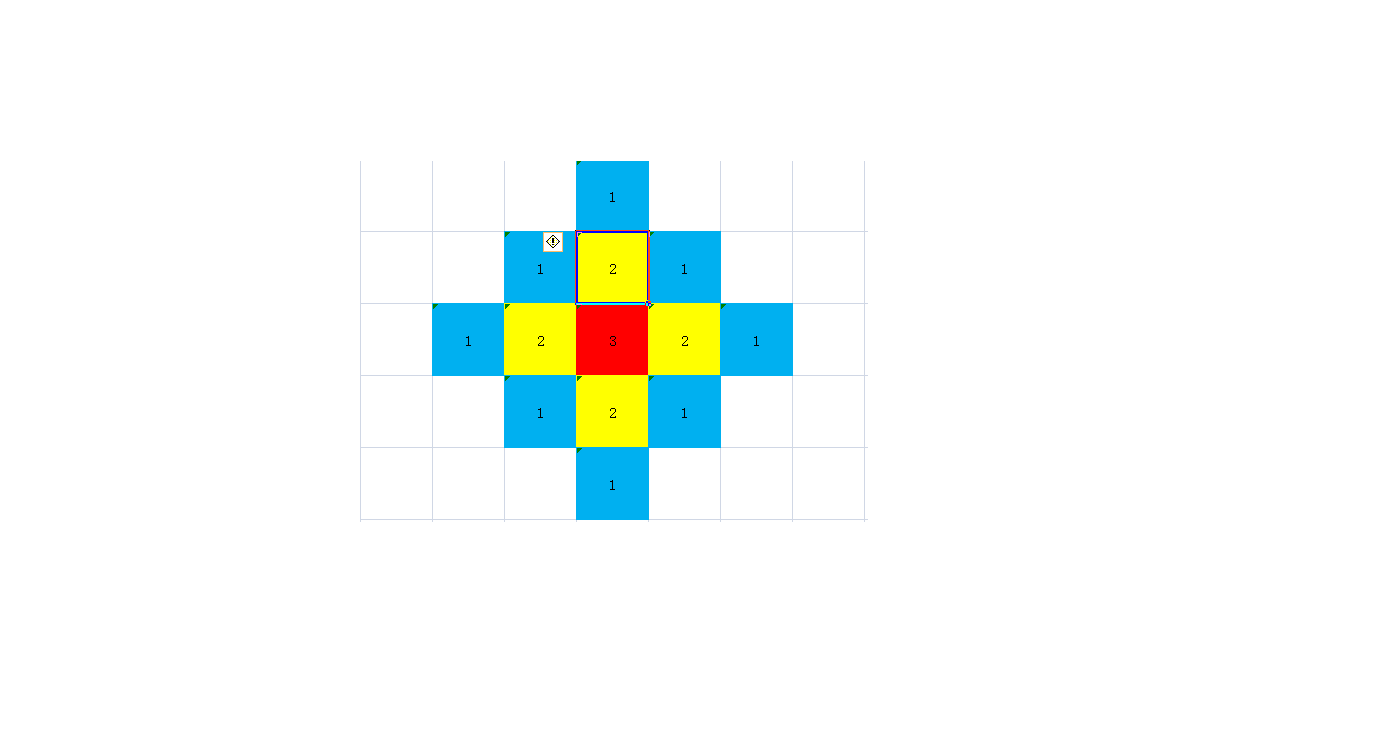# 【CSP-S膜你考】即时战略（模拟）

## Problem B. 即时战略 (rts.c/cpp/pas)

### 注意

Input file: rts.in
Output file: rts.out
Time Limit : 2 seconds
Memory Limit: 512 megabytes

### 题面

$\text{HLY}$在玩一个即时战略$\text{(Real Time Strategy)}$游戏。与大多数同类游戏类似，这个游戏的地图是平面的，并且玩家都有一个基地。

$\text{HLY}$的对手杰哥的基地是一个$w \times h$的矩形。其中矩形的每个格子都有一个建筑，每个建筑都有一个重要度。其中第$i$行第$j$列的格子中的建筑的重要度为$w_{ij}$

$\text{HLY}$决定轰炸杰哥的基地。他可以选择杰哥基地的任何一个格子释放一个能量为$p$的炸弹。释放以后，这个格子的建筑会受到$p$的摧毁度。炸弹产生的冲击波可以向四个方向扩散，每扩散一格能量值会减少$1$。即释放位置相邻的$4$个格子会受到$p − 1$的摧毁度，再向外的$8$个格子会受到$p − 2$的摧毁度 ... 直到能量值减为 $0$ ，形式化的讲，如果在第 $x$ 行第 $y$ 列释放炸弹，那么第 $i$ 行第$j$ 列的格子受到的摧毁度 $d_{ij} = max(0,p − (| x − i | + | y − j |))$。

$\text{HLY}$想知道，对于每一种投放炸弹的方案，杰哥受到的最小总损失和最大总损失各为多少，形式化的讲，即为

$\large \sum_{i=1}^{w}\sum_{j=1}^{h} w_{ij} \times d_{ij}$

### 输入格式

$1$行三个整数$w,h,p$

### 样例

$\texttt{input\#1}$
3 4 3
9 9 9 1
9 9 1 1
9 1 1 1

$\texttt{output\#1}$
10 96

### 数据范围与提示

$\text{HLY}$在第$2$行第$2$列释放炸弹杰哥所受损失最大，为
$9 \times 1 + 9 \times 2 + 9 \times 1 + 9 \times 2 + 9 \times 3 + 1 \times 2 + 1 \times 1 + 9 \times 1 + 1 \times 2 + 1 \times 1 = 96$
$\text{HLY}$在第$3$行第$4$列释放炸弹杰哥所受损失最小， 为
$1\times1+1\times1+1\times2+1\times1+1\times2+1\times3 = 10$

### 题解

$1 \le n,m \le 400$模拟可过，不过需要点技巧——前缀和。### $Code$

#include<iostream>
#include<cstring>
#include<string>
#include<cstdio>
#include<algorithm>
#define max(a,b) a>b?a:b
#define min(a,b) a<b?a:b
#define MAXN 2020
#define int long long

int w,h,p,ans1=0,ans2=1e18,map[MAXN][MAXN];
int sum1[MAXN][MAXN],sum2[MAXN][MAXN];

int x=0;bool f=0;char c=getchar();
while(c<'0'||c>'9'){if(c=='-')f=!f;c=getchar();}
while(c>='0'&&c<='9'){x=x*10+c-'0';c=getchar();}
T=f?-x:x;
}

signed main() {
w+=500,h+=500;//防止越界
for(int i=501;i<=w;++i) {
for(int j=501;j<=h;++j) {
}
}
for(int i=1;i<=2010;++i) {
for(int j=1;j<=2010;++j) {
sum1[i][j]=sum1[i-1][j-1]+map[i][j];
}
}//一个从左上到右下的前缀和
for(int i=1;i<=2010;++i) {
for(int j=2010;j>=1;--j) {
sum2[i][j]=sum2[i-1][j+1]+map[i][j];
}
}//一个从右上到左下的前缀和
for(int i=501;i<=w;++i) {
for(int j=501;j<=h;++j) {//枚举将炸弹放到哪一个点
int sum=p*map[i][j];
int x=p;
while(x>1) {
sum+=(p-x+1)*(sum1[i][j+x-1]-sum1[i-x][j-1]);
sum+=(p-x+1)*(sum1[i+x-1][j]-sum1[i-1][j-x]);
sum+=(p-x+1)*(sum2[i][j-x+1]-sum2[i-x][j+1]);
sum+=(p-x+1)*(sum2[i+x-1][j]-sum2[i-1][j+x]);
sum-=(p-x+1)*map[i-x+1][j];
sum-=(p-x+1)*map[i+x-1][j];
sum-=(p-x+1)*map[i][j-x+1];
sum-=(p-x+1)*map[i][j+x-1];
--x;
}//计算放到该点的摧毁度
ans1=max(ans1,sum);
ans2=min(ans2,sum);
}
}
std::cout<<ans2<<" "<<ans1<<'\n';
return 0;
}

posted @ 2019-10-13 21:25  yu__xuan  阅读(136)  评论(4编辑  收藏